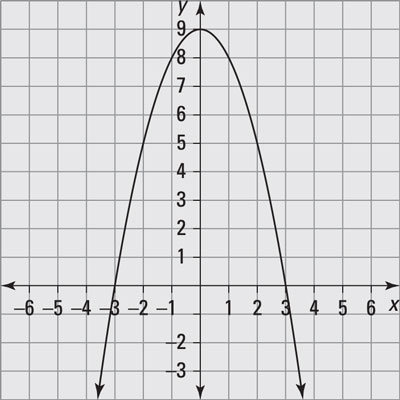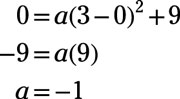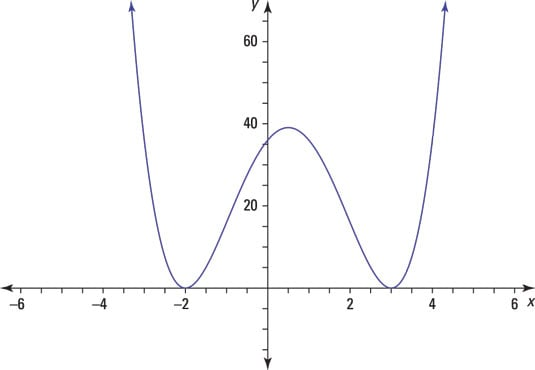##### Pre-Calculus: 1001 Practice Problems For Dummies (+ Free Online Practice)You can graph functions fairly handily using a graphing calculator, but you’ll be frustrated using this technology if you don’t have a good idea of what you’ll find and where you’ll find it. You need to have a fairly good idea of how high or how low and how far left and right the graph extends.

You get information on these aspects of a graph from the intercepts (where the curve crosses the axes), from any asymptotes (in rational functions), and, of course, from the domain and range of the function. A good working knowledge of the characteristics of different types of functions goes a long way toward making your graphing experience a success.

Another way of graphing functions is to recognize any transformations performed on basic function definitions. Just sliding a graph to the left or right or flipping the graph over a line is a lot easier than starting from scratch.

You’ll work with function graphs in the following ways:

• Graphing both a function and its inverse

• Determining the vertices of quadratic functions (parabolas)

• Recognizing the limits of some radical functions when graphing

• Pointing out the top or bottom point of an absolute-value function graph to establish its range

• Solving polynomial equations for intercepts

• Writing equations of the asymptotes of rational functions

• Using function transformations to quickly graph variations on functions

When graphing functions, your challenges include the following:

• Taking advantage of alternate formats of function equations (slope-intercept form, factored polynomial or rational functions, and so on)

• Determining whether a parabola opens upward or downward and how steeply

• Graphing radical functions with odd-numbered roots and recognizing the unlimited domain

• Recognizing when polynomial functions don’t cross the x-axis at an intercept

• Using asymptotes correctly as a guide in graphing

• Reflecting functions vertically or horizontally, depending on the function transformation

## Practice problems

1. Given the graph of a quadratic function, write its function equation in vertex form, y = a(xh)2 + k.Credit: Illustration by Thomson Digital

Answer: y = –x2 + 9

The vertex is (0, 9), and the graph opens downward. Using the vertex form of a quadratic equation, y = a(xh)2 + k, these characteristics are represented by y = a(x – 0)2 + 9, where a is a negative number.

The x-intercepts are (‒3, 0) and (3, 0). Substitute (3, 0) into the equation and solve for a:So the equation of the parabola is y = –1(x – 0)2 + 9.

2. Determine the intercepts of the graph of the polynomial. Then sketch the graph.

f(x) = (x – 3)2(x + 2)2

Answer: intercepts: (3,0), (‒2,0), (0, 36)

Find the x-intercepts by letting y = 0 and solving for x. The x-intercepts of y = (x – 3)2(x + 2)2 are (3, 0) and (‒2, 0).

Find the y-intercept by letting x = 0 and solving for y. The y-intercept is (0, 36).

To sketch the graph, note that the exponents on the factors are even numbers, so the curve just touches the x-axis at each x-intercept. The curve rises to the right as x approaches positive infinity, as determined when you test an x value greater than the right-most intercept. Here’s the graph:Credit: Illustration by Thomson Digital